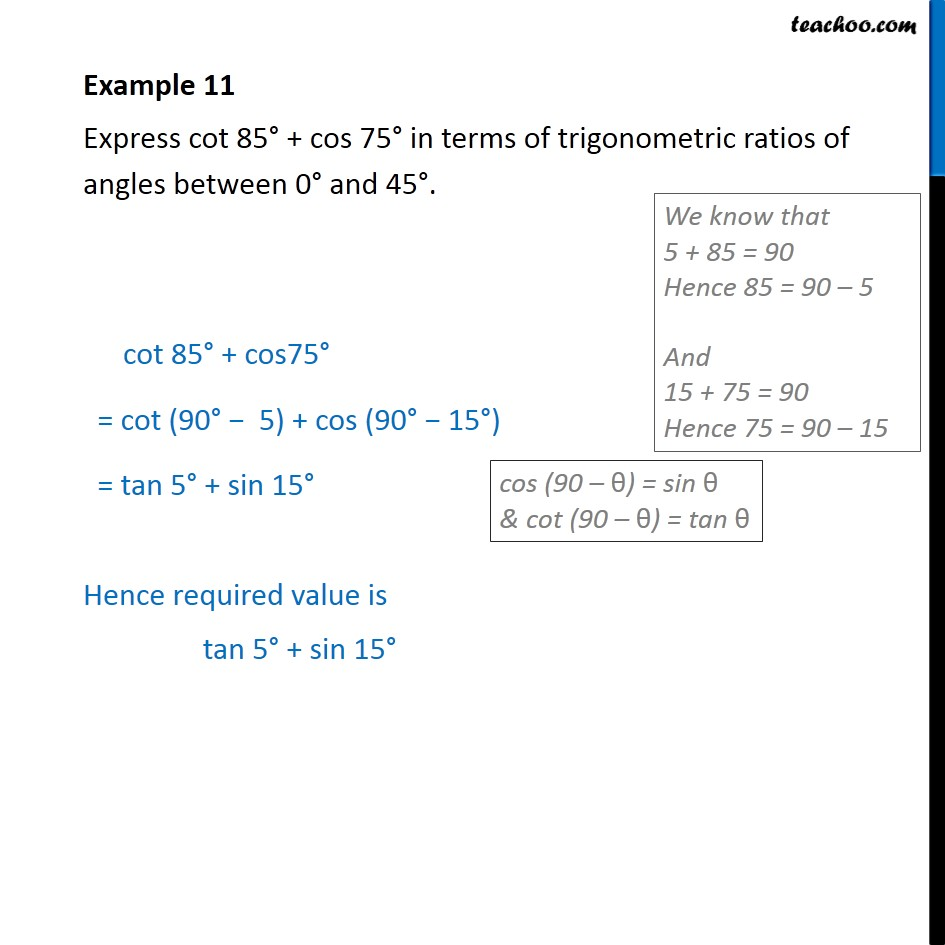1. Chapter 8 Class 10 Introduction to Trignometry (Term 1)
2. Serial order wise
3. Examples

Transcript

Example 11 Express cot 85° + cos 75° in terms of trigonometric ratios of angles between 0° and 45°. cot 85° + cos75° = cot (90° − 5) + cos (90° − 15°) = tan 5° + sin 15° Hence required value is tan 5° + sin 15°